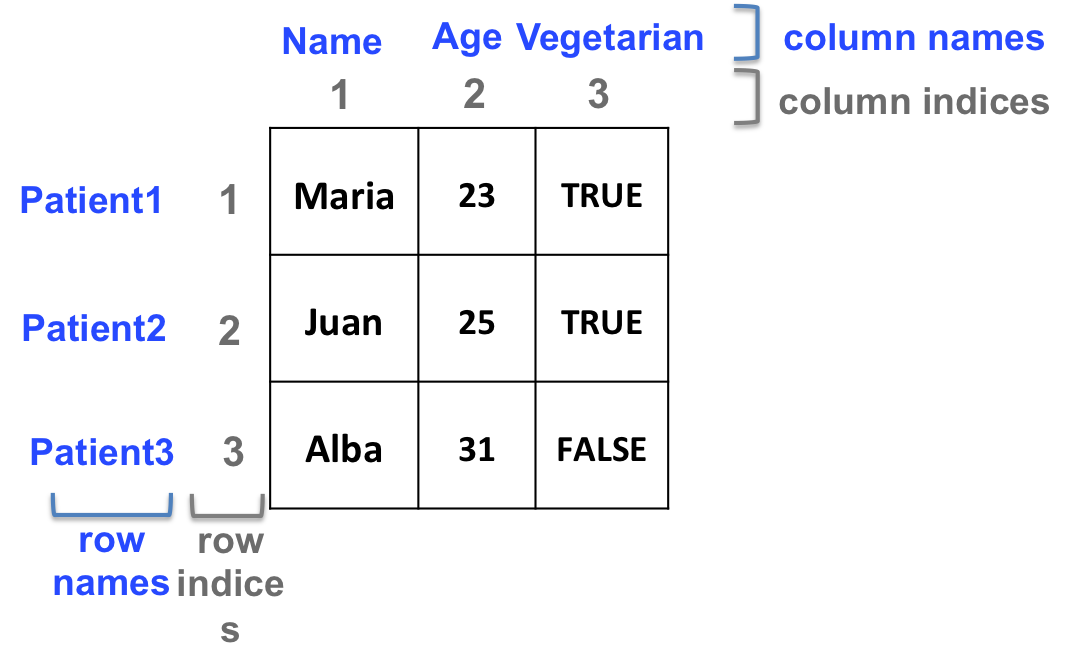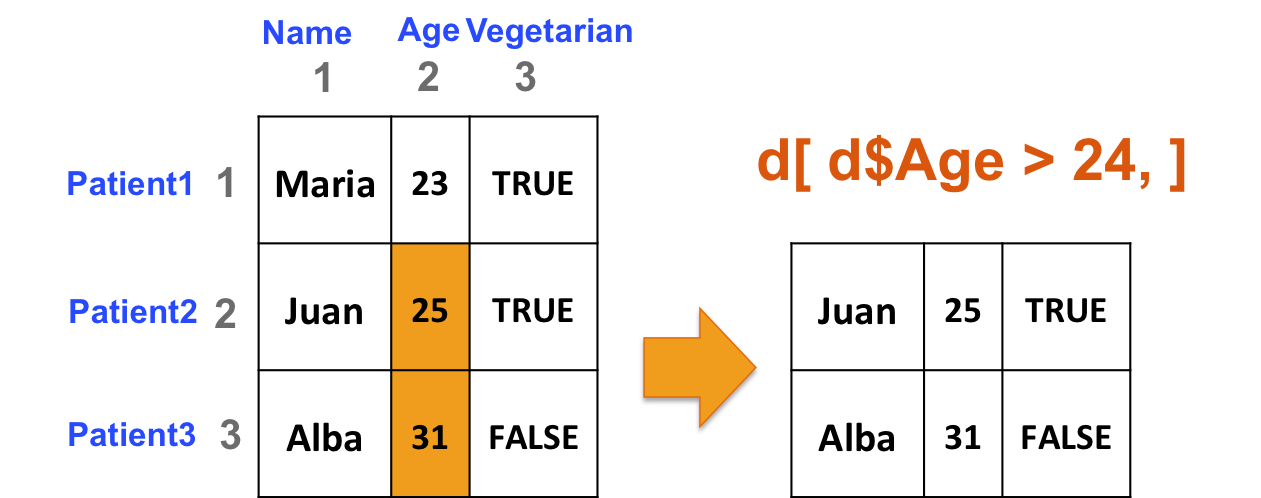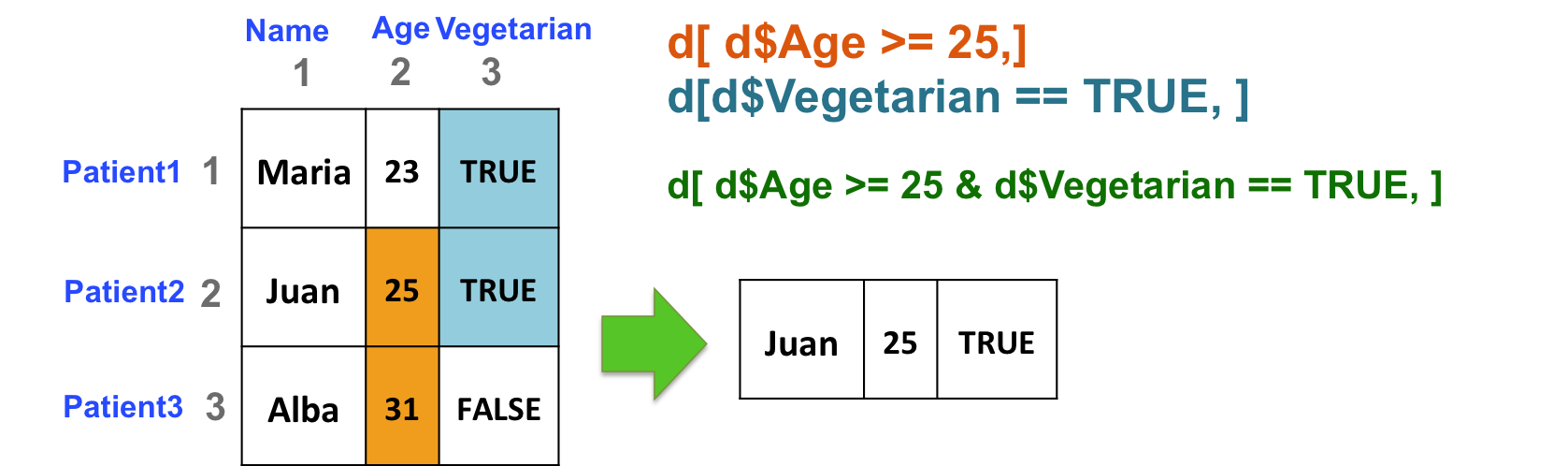## 9.7 Two-dimensional structures manipulation

### 9.7.1 Dimensions

• Get the number of rows and the number of columns:
``````# Create a data frame
d <- data.frame(c("Maria", "Juan", "Alba"),
c(23, 25, 31),
c(TRUE, TRUE, FALSE))
# number of rows
nrow(d)
# number of columns
ncol(d)``````
• Check the dimensions of the object: both number of rows and number of columns:
``````# first element: number of rows
# second element: number of columns
dim(d)``````
• Dimension names

Column and/or row names can be added to matrices and data frames

``````colnames(d) <- c("Name", "Age", "Vegetarian")
rownames(d) <- c("Patient1", "Patient2", "Patient3")``````

Column and/or row names can be used to retrieve elements or sets of elements from a 2-dimensional object:``````d[,"Name"]
# same as:
d[,1]

d["Patient3", "Age"]
# same as:
d[3,2]

# for data frames ONLY, the \$ (dollar) sign can be used to retrieve columns:
d\$Name
# is the same as
d[,"Name"]
# and the same as
d[,1]``````
• Include names as you create objects:
• Matrix:
``````m <- matrix(1:4, ncol=2,
dimnames=list(c("row1", "row2"), c("col1", "col2")))``````
• Data frame:
``````df <- data.frame(col1=1:2, col2=1:2,
row.names=c("row1", "row2"))``````

### 9.7.2 Manipulation

Same principle as vectors… but in 2 dimensions!

#### 9.7.2.1 Examples

• Select rows of b if at least one element in column 2 is greater than 24 (i.e. select patients if they are older than 24):
``````# build data frame d
d <- data.frame(Name=c("Maria", "Juan", "Alba"),
Age=c(23, 25, 31),
Vegetarian=c(TRUE, TRUE, FALSE))
rownames(d) <- c("Patient1", "Patient2", "Patient3")

# The following commands all output the same result:
d[d[,2] > 24, ]
d[d[,"Age"] > 24, ]
d[d\$Age > 24, ]

# Step by step:
# 1. "isolate" column of interest (here, the second column: "Age")
d[,"Age"]
# 2. apply your criterion (here, "Age" should be greater than 24)
d[,"Age"] > 24
# 3. Extract the corresponding rows from the original data frame
d[ d[, "Age"] > 24, ]``````• Select patients (rows) based on 2 criteria: age of the patient (column 2) should be great than or equal to 25, and the patient should be vegetarian (column 3):
``d[ d\$Age >= 25 & d\$Vegetarian == TRUE, ]``#### 9.7.2.2 Add rows and columns to matrices and data frames

• For matrices: add a row or a column with rbind and cbind, respectively:
``````# add a column
cbind(d, 1:3)
rbind(d, 4:6)``````
• Add columns (variables) to data frames:
``````# add column "Gender"
dgender <- data.frame(d, Gender=c("F", "M", "F"))``````
• Add rows (observations) to data frames:

As data frames columns can be of different type, rbind will not give the expected results.
Remember str()?
Let’s try to add a patient to our data frame d with rbind, and a new vector:

``````drb <- rbind(d, c("Jordi", 33, FALSE))
# check the structure
str(drb)
# what is the problem here?``````

Add a patient to d using data.frame: need to use an intermediate data frame, and not a vector:

``````# intermediate data frame
newd <- data.frame("Jordi", 33, FALSE)
# name the intermediate data frames columns the same as d's names
colnames(newd) <- colnames(d)
ddf <- rbind(d, newd)
# check the structure
str(ddf)``````

#### 9.7.2.3 Process the sum of all rows or all columns with rowSums and colSums, respectively.

``````# create a matrix
b <- matrix(1:20, ncol=4)
# process sum of rows and sum of cols
rowSums(b)
colSums(b)``````

#### 9.7.2.4 Check the first and last rows with head and tail:

``````# create a 5000 row matrix
bigmat <- matrix(1:20000, ncol=4)
# check the first and last 6 rows (by default)
tail(bigmat)
# check the first and last 10 rows
tail(bigmat, n=10)``````

HANDS-ON

Given the following data frame mypatients:

``````mypatients <- data.frame(Name=c("Maria", "Juan", "Alba", "Luis", "Hector"),
Age=c(23, 25, 31, 18, 42),
Weight=c(65, 72, 58, 78, 82),
Vegetarian=c(TRUE, TRUE, FALSE, FALSE, TRUE))``````
1. What is the average age of vegetarian patients?
2. Add a “Gender” column to mypatients (that contains “Female” and “Male” entries).
3. What is the average weight of vegetarian men?

#### 9.7.2.5 The apply function

Powerful tool to apply a command to all rows or all columns of a data frame or a matrix.
For example, instead of calculating the sum of each row, you might be interested in calculating the median ? But rowMedians doesn’t exist !

apply takes 3 arguments:

• first argument X: 2-dimensional object
• second argument MARGIN: apply by row or by column?
• 1: by row
• 2: by column
• third argument FUN: function to apply to either rows or columns
``````# median value of each row of b
apply(X=b, MARGIN=1, FUN=median)
# median value of each column of b
apply(X=b, MARGIN=2, FUN=median)``````

NOTE: apply is part of a family of functions. For more information, check the dedicated DataCamp chapter.

HANDS-ON

Given the following matrix datam:

``````# rnorm produces random numbers (here, 10000), from the normal distribution.
# you can set the "seed" so as to be able to exactly reproduce the random selection of numbers:
set.seed(43)
datam <- matrix(rnorm(10000), ncol=25)``````
1. How many rows does datam have?
2. Calculate the standard deviation of each column of datam (use apply and sd).
3. Calculate the median of each row of datam (use apply and median).

#### 9.7.2.6 Select rows or columns based on a pattern

Here we introduce the grep function, that can help you select rows and columns based on patterns.

``````# example data frame
dfgrep <- data.frame(genes=c("CCNA1", "TP53", "CCND1", "CCNF", "A2M", "PARP2", "TP63", "DKK1"),
log2FC_1=c(-2, -1, 0.5, 1.2, -5, 3.2, 1.3, 0.5),
pvalue_1=c(0.01, 0.4, 1, 0.9, 0.003, 0.96, 0.002, 0.67),
log2FC_2=c(0.1, -2, 3.5, -1.3, 1.5, 2, 2.1, -0.05),
pvalue_2=c(0.87, 0.06, 0.001, 0.4, 0.8, 0.76, 0.08, 0.99))``````
• Select the “pvalue” columns of dfgrep with grep:
``````# select the columns which names match "pvalue" (it does NOT have to be an exact match):
grep(pattern="pvalue", x=colnames(dfgrep))
# extract only those columns:
dfgrep[, grep(pattern="pvalue", x=colnames(dfgrep))]``````
• Select all tumor protein gene (TP genes) rows with grep:
``dfgrep[grep(pattern="TP", x=dfgrep\$genes), ]``

For more details about grep and pattern selection, you can refer to the dedicated extra section

• Useful grep arguments:

Show the selected names (not the indices):

``````# shows the indices of selected elements, by default:
grep(pattern="TP", x=dfgrep\$genes)
# shows the actual selected elements
grep(pattern="TP", x=dfgrep\$genes, value=TRUE)``````

Show what does NOT match:

``````# shows what matches the search:
grep(pattern="TP", x=dfgrep\$genes)
# shows what doesn't match the search:
grep(pattern="TP", x=dfgrep\$genes, invert=TRUE)``````

HANDS-ON

Let’s take the dfgrep data frame again:

1. What is the average “log2FC_1” and “log2FC_2” of the cyclin genes (CCN symbols)? Proceed this way:
• Select all CCN gene rows.
• Select all “log2FC” columns.
• Using the apply function, calculate the average of each “logFC” columns.